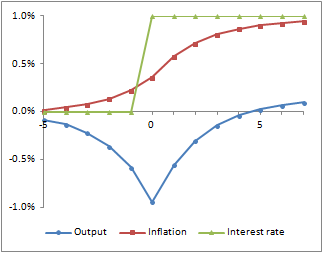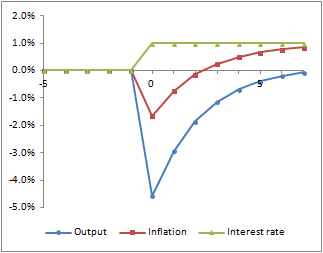## Thursday, 26 November 2015

### International Balances and the Exchange Rate

I've read a few things recently on the complex relationships between interest rates, exchange rates and trade balances.

I like to approach this by starting with the stock variables.  We can imagine a simple global balance sheet matrix like that set out below.  This looks at the position of Country X, which uses Currency X (and we'll assume for simplicity that only Country X uses Currency X).  The assets in other currencies are converted into Currency X at prevailing spot rates, so that we can do some adding up.

So at a particular point in time, investors of Country X hold total assets of 100, which they need to allocate.  Being based in Country X, there will be some preference for investing in currency X assets.  However, those investors will probably also wish to hold some level of other assets to diversify their portfolio.  The balance will depend to some extent on potential returns in different currencies, with the return being a function of nominal interest rates in the respective currencies and potential exchange rate movements.

 Currency X Assets Other Currency Assets Total Country X Investors 80 20 100 Rest of World Investors 50 850 900 Total 130 870 1,000

Likewise, investors in the rest of the world will also want to diversify their investments, so they will want to include some Currency X assets in their portfolio.  Again they will take potential returns into account.  There will be many other factors, of course, effecting what assets investors choose to hold.  But, all other things being equal, the better the prospects for returns in a currency, the more of it investors will want to hold.

Just as Country X investors have a preference for investing in their own currency, so will investors in the rest of the world.  So, the greater the share of total investments held by Country X investors, the greater the overall demand for Currency X assets.

This is what determines exchange rates in the very short term.  At any point in time, the amount of assets in each currency is given, as is the assets of each investor class.  Taking interest rates as given, the exchange rate must find a level where investors want to hold the assets that are available in each currency.

Generally, if there are more Currency X assets than investors wish to hold, then Currency X needs to become cheaper and it will depreciate until it finds the right level.  However, since the right level depends on potential future movements of the currency, which in turn depends on what the exchange rate will be in the future, this can be hard to pin down.  I will come back to this.

So far we have said nothing about trade flows.  In fact, current account items do not matter in themselves.  There is no need for the current account to balance in either the short or the long term.  The relevance of trade flows and investment income is that, over time, they change the balance of assets.

So, if country X is running a persistent current account deficit, then there will be corresponding changes in our matrix.  This could be reflected in a growth of Currency X assets, as Country X finances its spending with borrowing, or it might be reflected in a reduction in the total assets of Country X investors (or some combination of the two).

The impact depends on what is going on with investors and assets in the rest of the world.  In combination, these changes may result in the ratios of values within the matrix remaining relatively stable.  However, they could lead to significant changes.  For example, Country X's persistent deficits might lead to Currency X assets becoming a greater and greater proportion of the whole.  In that event we might expect to see some consequences for interest rates and/or exchange rates.

We can see what is happening with interest rates, but we are left with the question of what drives expectations of exchange rate movements.  If today's exchange rate falls, we might hazard a guess that it is more likely to return gains in the future, but it all depends on where the exchange rate needs to be going into the distant future.  We seem to need some kind of target exchange rate to which the actual exchange rate will move over time.

In some models, this target exchange rate role is filled by a measure of purchasing power parity.  The framework I'm using here suggests a slightly different approach.  What it requires is that the exchange rate moves towards a level that allows the values in the matrix to remain in balance.  In a growth scenario, this would mean all the values ultimately growing at the same rate.  Any exchange rate that fails to meet this criterion will lead to an unsustainable position of ever diverging values.

We can write down models that reflect this.  By making certain assumptions about behaviours, we can trace out exchange rate paths that tend towards these steady state positions, while fulfilling relative return requirements along the way.  But it should be noted that there are many factors coming into play in these models, not least how fiscal and monetary policy are expected to develop into the future.  However clearly the equilibrium exchange rate might be defined under perfect foresight, the reality of exchange rate determination is probably closer to blind guesswork.

 I have assumed here that Country X borrowers only borrow in Currency X, but in reality they might borrow in different currencies.  The same issues apply, but this explanation is much simpler if we ignore that.  On the whole, it is much less common for an entity to borrow (other than on a matched basis) outside its functional currency than it is to invest.

## Sunday, 15 November 2015

### Neo-Fisherism and Term Debt

I just got round to having a look at John Cochrane's recent paper on the neo-Fisherian hypothesis, that higher nominal interest rates raise inflation.  A main theme of this paper is the issue of whether there is a theoretical basis for thinking that, even if this holds as a long-run equilibrium condition, the short run impact is the reverse.  It is commonly believed that the initial response to a higher interest rate will be lower inflation; Cochrane looks at whether we are right to think this.

The paper makes a good case that the basic New Keynesian model does not in fact imply the conventional result.  Cochrane also looks at several possible variations but concludes that these do not providing a convincing case for an initial deflation.

In my view, there is a very simple reason explanation of why we might expect an initial deflation and it's to do with term debt.  This is easy to see within Cochrane's model.

He starts with two of the three equations from the basic New Keynesian model: an equation for output (y) based on the nominal interest rate (i) and the inflation rate (π) and a NK Phillips curve.

yt = E[yt+1] { β ( 1 + it ) / ( 1 + E[πt+1] ) }

1 + πt = ( 1 + E[πt+1] )β ytκ

The third equation (the central bank reaction function) is replaced with an interest rate peg.

Cochrane also considers the inclusion of a public sector budget constraint:

Bt = ( Bt-1 -  st pt ) ( 1 + it )

where B is the nominal stock of single period government debt by face value (which we'll call bills), s is the real primary public sector surplus and p is the price level.  This accounting identity can be extrapolated to show that the current real value of public debt is equal to the discounted value of future primary surpluses.  In turn, taking the historical level of public debt together with expected future surpluses and real interest rates gives us a current equilibrium price level, as follows:

pt = Bt-1 / E[St]

where E[S] is the expected present value of future surpluses.

Cochrane uses this to distinguish between the multiple equilibria that are admitted by the simple two equation model, but finds that this does not convincingly point to an initial deflation.

From looking at how SFC models work, the thing that I would do at this point is try to incorporate some term debt.  The easiest way to do that here is to suppose that some part of the public debt consists of an annuity which pays a fixed nominal amount.  The public sector budget constraint (assuming no new issue of annuities) is now:

Bt = ( Bt-1 + a -  st pt ) ( 1 + it )

where a is the nominal value of the annuity payment.  The equilibrium price level now becomes:

pt = ( Bt-1 + At  + a ) / E[St]

where A is the nominal present value of the future annuity stream based on expected future interest rates (and assuming here that this is valued and discounted at the same rate as is paid on bills).

Examining this formula, we can see why a rise in expected nominal interest rates might create an initial deflationary effect.  Whilst the interest rate change has no impact on the value of bills, it does effect the value of the annuity.  A rise in expected nominal interest rates reduces the value of the annuity and therefore reduces the equilibrium price level.  If annuities make up a sufficient part of the public debt, this results in an initial deflation.

The charts below show what this looks like in Cochrane's model.  I have used the same parameters and the charts are the equivalent of Cochrane's Figure 1, but with 25% by value of public debt assumed to be in the form of annuities.

The first chart shows the result of an expected increase in the nominal rate.  This chart is essentially the same as Cochrane's Figure 1.  This is what we would expect, because if everything pans out in line with expectations, there is no difference between bills and annuities.The second chart shows the impact of an unexpected rate increase.  This is very different.  Not only do we get a much greater fall in real output but we now also get a temporary fall in inflation.  This is the result of the shock revaluation of term debt.Of course, this result should be of no surprise to anyone familiar with SFC modelling.  These dynamics are typical of what happens in models which combine long run and short run debt and are relatively insensitive to assumptions about expectation formation or inter-temporal preference.

[Edited to conform a bit more closely to Cochrane.]